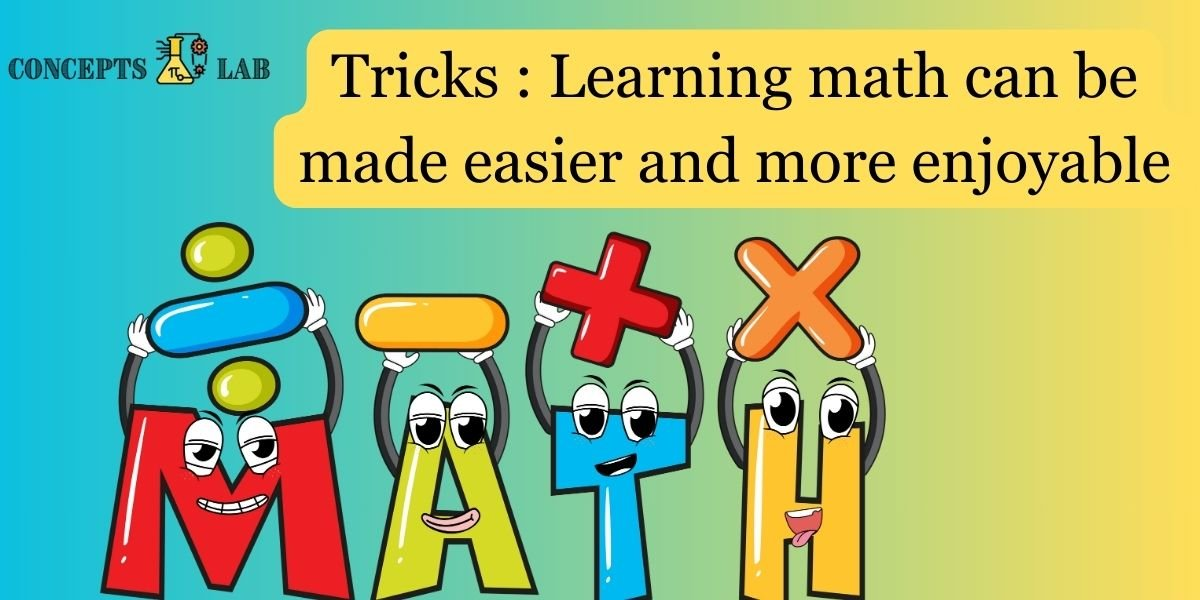Learning math can be made easier and more enjoyable with the help of various tricks and strategies. Here are some tricks to learn math with ease:

### 1. Understand the Basics Well:

• Ensure a strong foundation in basic arithmetic operations (addition, subtraction, multiplication, division). These are the building blocks for more complex math concepts.

### 2. Visualize Concepts:

• Use visual aids, diagrams, and illustrations to understand mathematical concepts. Visualizing problems can make them more tangible and easier to solve.

### 3. Practice Regularly:

• Practice is key to mastering math. Regularly solve problems and exercises to reinforce concepts. Consistent practice helps build confidence.

### 4. Break Down Problems:

• Break complex problems into smaller, more manageable steps. Focus on solving each step before moving on to the next.

### 5. Create Mnemonics:

• Use mnemonics, acronyms, or memory aids to remember formulas, the order of operations, or other key concepts. Mnemonics make memorization more fun.

### 6. Use Real-Life Examples:

• Relate math problems to real-life situations. This makes abstract concepts more relatable and helps in understanding their practical applications.

### 7. Math Games and Apps:

• Explore educational math games and apps. Many apps are designed to make learning math enjoyable and interactive.

### 8. Group Study:

• Study with classmates or friends. Explaining concepts to each other helps reinforce understanding, and group discussions can provide different perspectives.

### 9. Teach Someone Else:

• The best way to solidify your understanding is to teach someone else. It could be a friend, a family member, or even an imaginary friend. Teaching forces you to organize your thoughts.

10. Use Analogies:

``````- Draw parallels between mathematical concepts and things you find easy to understand. Analogies can make abstract ideas more concrete.

``````

Remember, everyone learns differently, so find the strategies that work best for you. The key is to approach math with a positive mindset and a willingness to explore various learning methods.